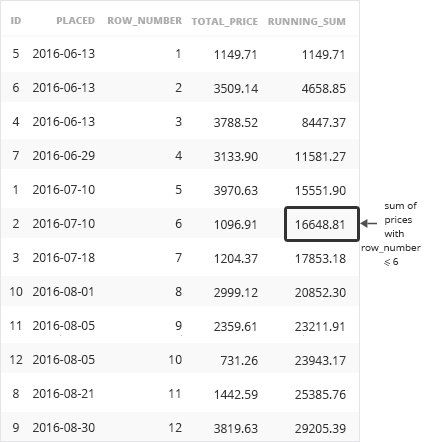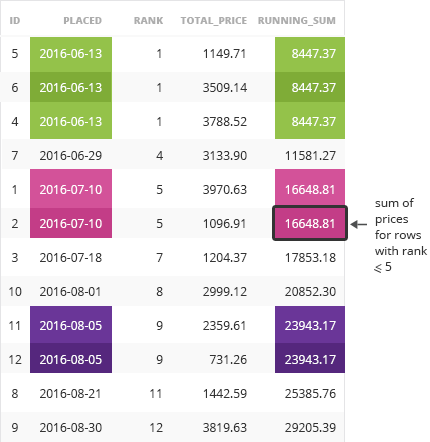Deals Of The Week - hours only!Up to 80% off on all courses and bundles.-Close
Introduction
ROWS
RANGE
14. ROWS and RANGE – explanation
Default window frame
Summary

## Instruction

The difference between ROWS and RANGE is similar to the difference between the ranking functions ROW_NUMBER and RANK()

The query with ROWS sums the total_price for all rows which have their ROW_NUMBER less than or equal to the row number of the current row.

SELECT
id,
placed,
total_price,
ROW_NUMBER() OVER(ORDER BY placed),
SUM(total_price) OVER(
ORDER BY placed
ROWS UNBOUNDED PRECEDING)
FROM single_orderThe query with RANGE sums the total_price for all rows which have their RANK() less than or equal to the rank of the current row.

SELECT
id,
placed,
total_price,
RANK() OVER(ORDER BY placed),
SUM(total_price) OVER(
ORDER BY placed
RANGE UNBOUNDED PRECEDING)
FROM single_order## Exercise

Click to continue